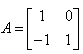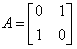Courses

# Test: Elementary Operations

## 5 Questions MCQ Test Mathematics (Maths) Class 12 | Test: Elementary Operations

Description
This mock test of Test: Elementary Operations for JEE helps you for every JEE entrance exam. This contains 5 Multiple Choice Questions for JEE Test: Elementary Operations (mcq) to study with solutions a complete question bank. The solved questions answers in this Test: Elementary Operations quiz give you a good mix of easy questions and tough questions. JEE students definitely take this Test: Elementary Operations exercise for a better result in the exam. You can find other Test: Elementary Operations extra questions, long questions & short questions for JEE on EduRev as well by searching above.
QUESTION: 1

### To check whether the matrix B is an inverse of matrix A, we need to check whether​

Solution:

An n×n matrix A is said to be invertible if there exists an n×n matrix B such that AB=I, and BA=I, where I is the n×n identity matrix.
If such a matrix B exists, then it is known to be unique and called the inverse matrix of A, denoted by A−1.

QUESTION: 2

### The inverse of a matrix is defined for

Solution:

The inverse exists only for square matrix. However this is not true. A nonsingular matrix must have their inverse whether it is square or nonsquare matrix.

QUESTION: 3

### The inverse ofis

Solution:

A = IA
AA-1 = I
A = {(1,0),(-1,1)}
A-1{(1,0),(-1,1)} =  {(1,0),(0,1)}
R2 ----> R2 + R1
A^-1 {(1,0),(0,1)} = {(1,0),(1,1)}
I A-1 = {(1,0),(1,1)}
Therefore, A-1 = {(1,0),(1,1)}

QUESTION: 4

The inverse ofis

Solution:

A = {(0,1) (1,0)}
|A| = (0 - 1) = -1
= -1{(0,(-1)) ((-1),0)}
= {(0,1) (1,0)}

QUESTION: 5

If a matrix B is obtained from matrix A by an elementary row or column transformation then B is said to be ______ of A​

Solution: